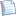# Review 2: Divide-and-conquerprintable version

R2:      

### Problem R2.1

In terms of n, the parameter, write a recurrence representing the amount of time taken by the below recursive algorithm. You can use variables C and D to represent constants that will vary from computer to computer.

def recur(n):     if n <= 1:         return n     else:         return recur(n - 1) + recur(n / 2)